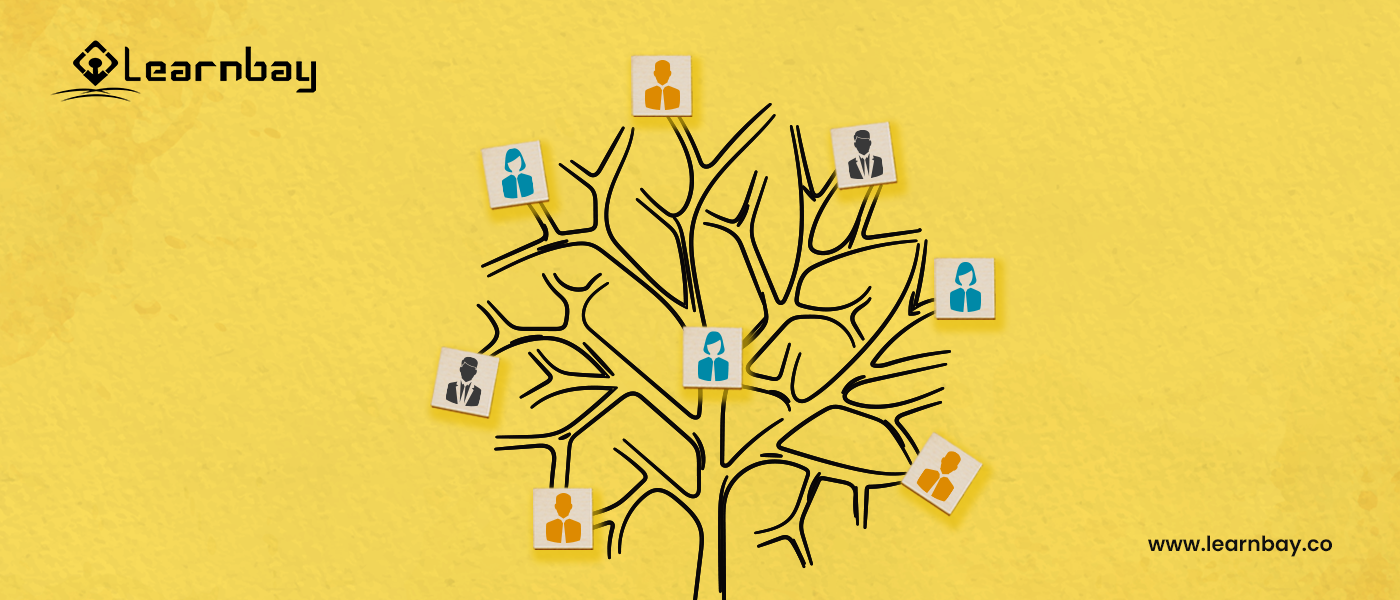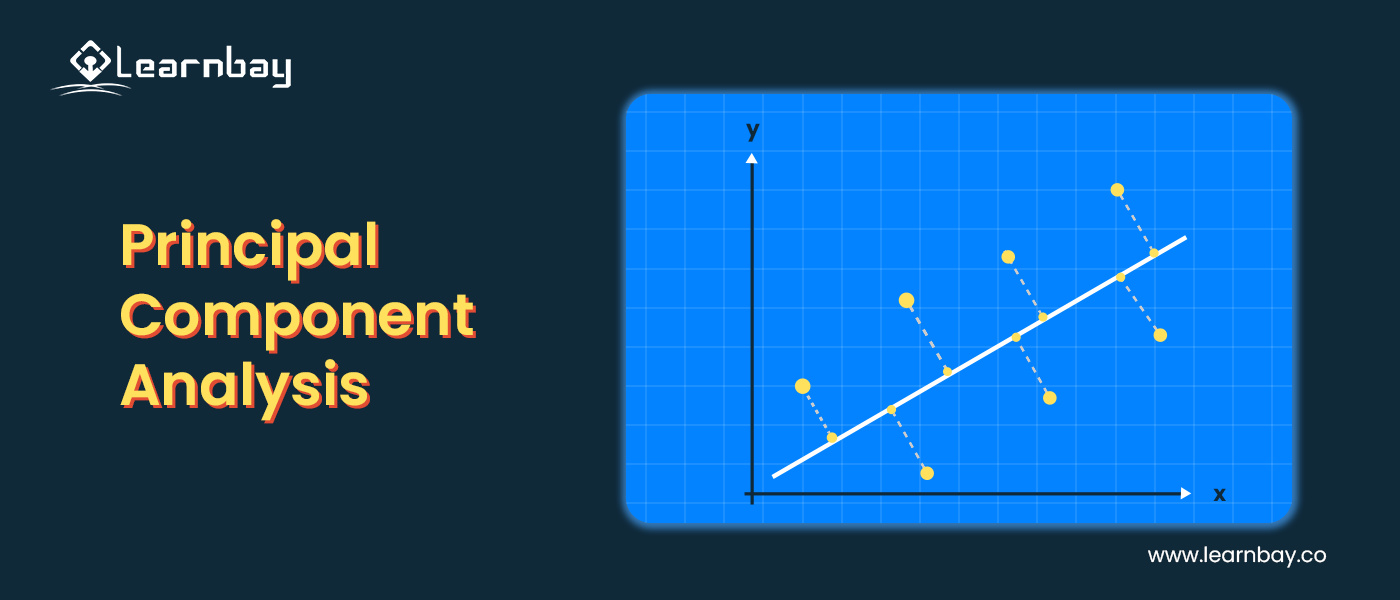# What is Supervised, Unsupervised Learning, and Reinforcement Learning in Machine Learning

By Learnbay Category Machine Learning Reading time 7-8 mins Published on Apr 7, 2022

Learn the Difference Between Supervised, Unsupervised, and Reinforcement Learning

If you want to understand how to utilize the massive amount of data presented to you effectively, then Machine learning will surely benefit you.

Machine learning (ML) models use algorithms and learn from datasets to understand and replicate human behavior. It is a branch of Artificial intelligence (AI). Therefore, it requires intelligent algorithms to be able to function efficiently.

There are different Machine learning models (Supervised, Unsupervised, and Reinforcement Learning) that are suitable for different kinds of datasets.

For example, supervised, unsupervised, and reinforcement learning in machine learning algorithms are widely used in industries to predict business outcomes. It helps professionals to forecast results based on historical data.

Let's understand it with an elaborate example.

You have a bucket of fruits. There are different types of fruits in the bucket. Now, you need to separate the fruits according to their features. Here, you know the name of the fruits and follow up on their corresponding features.

The features of the fruits are independent variables, and the name of the fruits are dependent variables; that is, the target variable. So you can build a predictive model to determine the fruit name.

## Supervised Machine Learning:-

The output of any supervised learning depends on the target variables. It allows the numerical, categorical, discrete, and linear datasets to build a Machine Learning model. The target variable is known for building the model. That model predicts the outcome based on the target variable if any new data point comes to the dataset.

The supervised learning model teaches the machine to predict the result for the unseen input. It contains a known dataset to train the machine and its performance during the training time of a model. And then, the model predicts the testing data's response when fed to the trained model. The supervised algorithm uses classification and regression techniques for building predictive models.

## Types of Supervised machine learning

### a. Linear regression & Logistic regression

Linear and logistic regression are used when we have continuous data. Linear regression defines the relationship between the variables where we have independent and dependent variables.

For example, what would be the performance percentage of a student after studying for several hours?

The number of hours is an independent feature , and the student's performance is the dependent feature.

Linear regression is also categorized into different types. Those are simple linear regression, multiple linear regression, and polynomial regression.#### b. Decision tree - Random forest & k-Nearest neighbors

Classification algorithms help to classify categorical values. It is used for categorical, discrete, or values belonging to a particular class. Decision tree, Random forest, and KNN are all used for the categorical dataset.

Popular or major classification applications include bank credit scoring, medical imaging, and speech recognition.

Also, handwriting recognition uses classification to recognize letters and numbers. Not only that, it further checks whether an email is genuine or spam. It also detects whether a tumor is benign or cancerous.

### c. Support vector machine

The support vector machine is used for both classification and regression problems. It uses the regression method to create a hyperplane to classify the category of the data point. Sentiment analysis of a subject is determined with the help of support vector machines whether the statement is positive or negative.

## Unsupervised Learning Algorithms

Unsupervised learning is a technique in which we need to supervise the model. Here, we do not have any target variable or labeled dataset. It discovers its information to predict the outcome. It is used for unlabeled datasets.

Unsupervised learning algorithms allow you to perform more complex processing tasks than supervised learning.

Although unsupervised learning can be more unpredictable compared with other machine learning methods, it is easier to get unlabeled data from a computer than labeled data, which needs manual intervention.

For example, We have a bucket of fruits and need to separate them accordingly. No target variable is available to determine whether the fruit is an apple, orange, or banana. Unsupervised learning categorizes these fruits to make a prediction when new data comes.

## Types of unsupervised learning

### a. Hierarchical clustering

Hierarchical clustering is an algorithm that builds a hierarchy of clusters. It begins with all the data assigned to a cluster of their own. Here, two close clusters are going to be in the same cluster. This algorithm ends when there is only one cluster left.

### b. K-means clustering & K-NN (k nearest neighbors)

K-means and KNN are also clustering methods to classify the dataset. K-means is an iterative method of clustering. It is used to find the highest value for every iteration. We can select the number of clusters. It would help if you defined the k cluster for making an excellent predictive model.

K-nearest neighbor is the simplest of all Machine Learning classifiers. It differs from other Machine Learning techniques because it doesn't produce a model. Instead, it is a simple algorithm that stores all available cases and classifies new instances based on a similarity measure.### c. Principal Component Analysis

PCA (Principal component analysis) is a dimensionality reduction algorithm. For example, you have a dataset with 200 features/columns. You need to reduce the number of features for the model to only an important feature. It maintains the complexity of the dataset.

### d. Singular Value Decomposition

A matrix's Singular Value Decomposition (SVD) in linear algebra is factoring that matrix across three matrices. It possesses several intriguing algebraic characteristics and provides essential geometrical and theoretical insights into linear transformations. It has several critical uses in data science as well. One of them is to help data scientists in getting the 'best fit' hyperplane, which segregates or categorizes the dataset under consideration.

### e. Independent Component Analysis

In various uses involving medical signals, biological testing, & audio signals, ICA (Independent Component Analysis) has been utilized to recover source signals. When ICA may eliminate or keep a single source, it is referred to as a dimensionality reduction algorithm.

## Reinforcement Learning

Reinforcement learning is one of the most utilized machine learning algorithms. Reinforcement learning is a machine learning programming strategy that rewards positive actions while penalizing undesirable ones.

A reinforcement learning agent, in general, can detect and comprehend its surroundings, act, and learn via trial and error.

It provides a suitable action in a particular situation and maximizes the reward. The reward could be positive or negative based on the object's behavior. Reinforcement learning is employed by various software and machines to find the best possible behavior in a given situation.

### Main points in Reinforcement learning

• Input: The input should be an initial state from which the model will start.
• Output: There are many possible outputs, as there are a variety of solutions to a particular problem.
• Training: The training is based on input. The model will return a state, and the user will decide to reward or punish the model based on its output.
• The model keeps learning.
• The best solution is decided based on the maximum reward.

## How does it work?

Developers establish a way of rewarding desired actions and punishing bad ones in reinforcement learning. This strategy provides positive values to desired acts and negative ones to undesirable behaviors to motivate the agent. The agent is programmed to find the long-term and greatest overall return to obtain an ideal answer.

These long-term objectives keep the agent from stagnating on short-term objectives. The agent gradually learns to shun the negative and pursue the positive. AI technology has implemented this learning strategy to control unsupervised machine learning via incentives and punishments.

## In Conclusion:-

Supervised learning is when a model is guided in learning from a labeled dataset. On the other hand, unsupervised learning is when the computer receives instruction based on unlabeled data with no direction. Reinforcement learning occurs when a system or perhaps an agent engages with its environment, takes actions, and learns via a trial-and-error process.

However, these were just a drop of water within a sea. An AI and ML program will help you understand these techniques in-depth through experienced mentors if you are interested in a detailed overview.